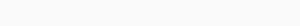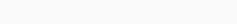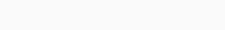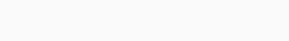# Geometric intuition for K-NN

In this section we will go through simpliest yet powerful classification technique called k-nn. So let’s see geometrically how k-nn works.Imagine we have 2 class of points, blue as positive data point and red as negative data point. The whole set of data points represent our dataset. What is the whole purpose of machine learning? we are given a dataset, our machine learning algorithm learn something from it. Now given a new query point to the same function, represented as yellow, it now says whether it is poitive or negative.What does K-NN do?

1. Finds K- nearest points to xq(query point) in our dataset (Let’s assume we are using distance as a measure to pick nearest point and k = 3  i.e picking 3 nearest point).
2. Let’s assume, {x1, x2, x3} as the 3 nearest neighbour to xq, now for each of the x’s in our set we have corresponding y’s that represent their class label i.e positive or negative.
3. Now we take the class label of the three points {y1, y2, y3}. Next we do a majority vote with the class label {y1, y2, y3}, whatever would be the majority vote for a class label that would be our class label for query point xq. Here, in this example, the majority of the class is positive, so our query point would be positive.

## Failure of K-NNCase 1

What if our query point is very very far away from our rest of the points? After taking 3 nearest neighbour, we might conclude that our query point is positive as majority points are positive, But would it be correct to call this query point as positive? So when the query point is far away from the datapoints, K-nn may not work. In this example as you can see, data is well organised, but still one far away point can cause chaos in the model. So this is one case where K- nearest neighbour may not work.Case 2

Now, imagine we have data points like this, randomly spreaded or in other words, jumbled up. We can’t conclude that our query point is positive or negative just on the basis of nearest neighbour and majority votes. There is no information here, we can’t declare anything from it.

## Distances

Since we started K-NN we are talking distance between points, So now let’s discuss about what is distance and it’s typesEuclidean distance

Let’s assume we have 2 points as x1 and x2 which are in 2-dimension, so our first dimension is feature 1(f1) and our second dimension is feature 2(f2). x1 can be represented as x11 and x22 and x2 can be represented as x21 and x22. Simple understanding of the distance is, shortest distance between the points i.e represented as d in the image. Now just by using simple pythagoras theoramWhen we put 2 vertical line around it, it means [length of].  ||x1– x2|| this distance is called Euclidean distance. Usually euclidean distance is represented as ||x1– x2||2 it is called L2 norm of vector.

Manhattan distance

In Euclidean distance as we used pythagoras theoram, but in manhattan distance we just use the sum of the distance (x21 – x11) +  (x22 – x12). When we put 1 vertical line around it, it means absolute. this distance is called Manhattan distance. Usually Manhattan distance is represented as ||x1– x2||1 it is called L1 norm of vector. where   ||x1– x2||1 is nothing but d=  |x1– x2

Minkowski distance

There is generalization to L1 norm and L2 norm known as Lp norms, and distance used for Lp norm is called Minkowski distance, formula used for this distance is d=  (|x1– x2|p)1/p where p can be any number, if you see this formula carefully, you may notice if p=2, formula becomes exact same as euclidean distance and if p=1, formula becomes same as manhattan distance

## Regression for K-NN

Until now we have saw K-nn for classification, data set for classification is represented aswhere xi are the data points, which are real valued numbers and yi’s are our class label.

So dataset for regression is where yi’s are not class label, but real valued numbers, it looks like following.STEPS for regression in KNN

1. For the given query point find the k-nearest neighbours(x1,y1),(x2,y2)…(xk,yk) (In classification what did we do? we took all the yi’s and did the majority vote).
2. But here, we will take all our yi’s (y1,y2,…yk) and this all yi’s are not class label, rather they are numbers, so we will take the mean or median(not more prone to outliers than mean) of this yi’s and resulting value would be our value for a query point.

## How to choose the right K

We have not yet discussed on how to pick the right K for our machine learning algorithm, K in K-nearest neighbour is a hyper parameter, we will discuss what is hyper parameter in upcoming topics, so let’s discuss how to pick right K and how our model changes as K changes.Imagine i have a dataset consisting of positive datapoints and negative data points, one thing you will notice here is, we have one negative data point in the region of positive points and one positive point in region of our negative pointsNow, if i ask you, can you separate positive points with negative points, then you will draw a curve that will look somewhat like this which is given in the image. Yes, there are 2 misclassifed points but the curve looks smooth and neat.Now again if i ask you to separate positive points and negative points, but you have a mindset of making no errors, curve will look like this in the image. Even though there are no missclassified points, but the curve is non smooth, there are twist and turn.

In the above 2 examples we draw curves, the curves that we draw to separate positive points and negative points is called decision surfaces.Let’s take value for K as 1, and  q1, q2, q3 be the query point and we want to find it’s class label. For a query point q1, the nearest point is positive point so it will be classified as positive point. For q2, nearest point is negative point so it will be classified as negative class. Now comes the fun part, what class label is appropriate for q3, as nearest point is negative point but it lies in the region of positive point, as we took the value for K as 1, q3 will be classified as negative point.If we took the value for K as 5, then our misclassified point q3 in our previous example would be classified as positive point, because we are going to take the majority vote and majority of the points that are around our query point q3 are positive points. For K=5 our curve would be smooth and for K=1 our curve would look twisted, So remember as our K increases, smoothness of the curve also increases.

Now think what will happen in K=n, where n is the maximum number K can have, and our dataset is imbalanced i.e 1000 positive points and 300 negative points, so as K=n, my query point will belong to positive class. n= postive points+negative points i.e 1300, and as there are just 300 negative points, our query point is 1300-NN so as 1000 points belongs to positive class, query point will be classified as positive according to majority vote.

When the value for K was 1, it was making no error, it was fitting every data points, curve was not smooth. That resulted in overfitting of the model as the two misclassified points in the data are either noise or outliers, K=1 was considering them as data, whichb resulted in overfitting, while value for K was 3, curve was smooth, and for a query point, the decision surface for K=5 worked well, so that resulted in well fitting of the model. And when K=n, query points class label was the class label that has dominance, which resulted in underfitting of the model.

## Space and Time complexity

For a given query point xq, how much time and space complexity is used to get yq . So how do we compute? for each datapoint xi in our dataset, we will first compute the distance between xi’s and query point xq and then store the k-smallest distance. Imagine we have n data points and each point is d-dimension. So for computing distance between xi’s and query point xq it will take order of d( O(d) ), and to store k-smallest distance it will take order of K( O(K) ), which is small. Now we have to compute this for n data points right. So the overall time complexity for K-nn is O(nd) we are neglacting complexity of O(K) because it is much much smaller. Space complexity for K-nn at evaluation time, i.e space that is needed to evaluate yq given xq is  O(nd) which is alot large, because of such large space complexity K-nn is not widely used for solving machine learning problem.

## Getting hands-on

We will be using Weka, an open source software to get hands-on experience of K-nearest neighbours. Before diving into code let’s understand what is weka. Weka is a collection of machine learning algorithms for data mining tasks. It contains tools for data preparation, classification, regression, clustering. Math over here would be taken care by WEKA. This is where you will learn more about it. https://www.cs.waikato.ac.nz/ml/weka/

## Let's get started

As we know, ARFF is the supporting file format used by weka, so we will be using Pima Indian diabetes dataset to understand K-nn and how our model performs as we change our K. We will be using dataset made available for us by weka  https://storm.cis.fordham.edu/~gweiss/data-mining/datasets.html

Before moving forward let’s understand the data, and what attributes does it hold. No. of instances our data have is 768 out of which 28 instances would be used by us for testing, resulting in 740 instances for training. There are all together 8 attributes and 1 class label.

Attributes information is as follows:
1. Number of times pregnant
2. Plasma glucose concentration a 2 hours in an oral glucose tolerance test
3. Diastolic blood pressure (mm Hg)
4. Triceps skin fold thickness (mm)
5. 2-Hour serum insulin (mu U/ml)
6. Body mass index (weight in kg/(height in m)^2)
7. Diabetes pedigree function
8. Age (years)
9. Class variable (0 or 1)

Class 1 represents: tested positive for diabetes. Class 0 represents: tested negative for diabetes

This is how are dataset will look:## Let’s dive into coding part

Let’s import some required library, loading our data set into memory and separate variable and class that we want to predict.

K-nn is also known as lazy classifier as it does not have any optimization, it just uses distance as a measure to classify instances. That’s why our K-nn is in weka.lazy package and K-nn is known as IBk.

				
import weka.core.converters.ConverterUtils
import weka.classifiers.lazy.IBk

val source = ConverterUtils.DataSource("diabetes.arff")
val traindataset = source.dataSet
traindataset.setClassIndex(traindataset.numAttributes() - 1)
val numClasses = traindataset.numClasses()
for (i in 0 until numClasses) {
val classvalue = traindataset.classAttribute().value(i)
println("Class Value " + i + " is " + classvalue)
}It’s time to build the classifier. we are going to use K as 4 in this example, we would be discussing later on how model changes when K changes.

				
val knn = IBk(4)
knn.buildClassifier(traindataset)



Next we will load our testing data and separate variable with class label.

				
val source1 = ConverterUtils.DataSource("diabetes_test.arff")
val testdataset = source1.dataSet
testdataset.setClassIndex(testdataset.numAttributes() - 1)



Now, let’s use our model that we build to check how it performed on testing data.

				
println("Actual, pred")
//num instance returns no. of instance of the dataset
for (i in 0 until testdataset.numInstances())
{
//Converting actual class value of test data to distinct value
val actualclass = testdataset.instance(i).classValue()
val actual = testdataset.classAttribute().value(actualclass.toInt())

//Using our classifier to predict test data class label and compare it with actual values
val newinst = testdataset.instance(i)
val pred = knn.classifyInstance(newinst)
val predstring = testdataset.classAttribute().value(pred.toInt())
// comparison of predicted and actual

println("$actual,$predstring")
}Let us check out how our model performed by evaluating it.

				
val eval = weka.classifiers.Evaluation(traindataset)
eval.evaluateModel(knn, testdataset)
print(eval.toSummaryString("\nResults\n======\n", false))We are having an accuracy of 70.37% not that bad based on the lazy model that we are using. Now let’s check out what happens when we change the value of K.

				
val knn=IBk(3)
knn.buildClassifier(traindataset)For K=3 our model gave accuracy of 66.6%

				
val knn=IBk(5)
knn.buildClassifier(traindataset)For K=5 our model gave the accuracy of 66.6%

				
val knn=IBk(7)
knn.buildClassifier(traindataset)For K=7 our model gave the accuracy of 62.96%

				
val knn=IBk(9)
knn.buildClassifier(traindataset)For K=9 our model gave the accuracy of 51.85%

Hence optimal K that resulted in good result was K=4, always remember trial and error is a big part of modelling in machine learning, scenario always changes according to the data that we have.

We now conclude the topic, “K-Nearest Neighbours”.

Thank you for spending time on this course! Hope you learned a lot.